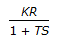# Chemical Engineering - Process Control and Instrumentation

### Exercise :: Process Control and Instrumentation - Section 5

26.

Gas analysis is commonly done using the

 A. thermal conductivity cell B. X-ray diffraction C. mass spectrometer D. emission spectrometer

Explanation:

No answer description available for this question. Let us discuss.

27.

The open loop transfer function of a control system is. This represents

 A. a first order system. B. dead time system. C. a first order time lag. D. a second order system.

Explanation:

No answer description available for this question. Let us discuss.

28.

Thermal conductivity measurement is used for the determination of

 A. O2 percentage in the flue gas. B. specific gravity of petrofuels. C. composition of an alloy. D. CO2 percentage in the flue gas.

Explanation:

No answer description available for this question. Let us discuss.

29.

Presence of a small amount of water in the organic systems can be determined by the __________ method.

 A. electrical conductivity B. polarimetry C. emission spectroscopy D. dielectric constant end loss factor

Explanation:

No answer description available for this question. Let us discuss.

30.

The transfer function of a pure dead time system with dead time τd is

 A. 1/(τds + 1) B. τds + 1 C. e-τss D. eτds